# Rayleigh distribution

(redirected from Reighley distribution)

## Rayleigh distribution

[′rā·lē ‚dis·trə‚byü·shən]
(statistics)
A normal distribution of two uncorrelated variates with the same variance.

## Rayleigh Distribution

The Rayleigh distribution of the probabilities of the random variable X is characterized by the probability density function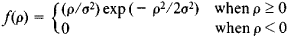The distribution function is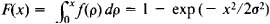The mathematical expectation is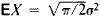and the variance is DX = (4 - π)σ4/2. The maximum value of the density function is equal to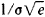and is reached when x= σ. Curves of the density function for various σ are shown in Figure 1.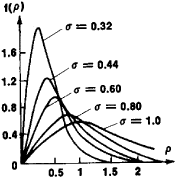Figure 1

The Rayleigh distribution is encountered in applications of probability theory to, for example, radio engineering. The distribution was introduced by Lord Rayleigh in 1880 in connection with the problem of interference of harmonic oscillations with spiral phases.

## Rayleigh distribution

(mathematics)
A curve that yields a good approximation to the actual labour curves on software projects.

Site: Follow: Share:
Open / Close